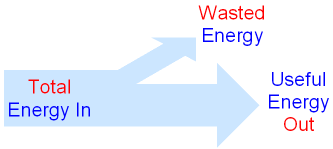gcsescience.com                                       20                                       gcsescience.com

Energy Transfer

What is Energy Efficiency?

Energy efficiency is a measure of how well
a device transfers energy into the form we want.

What is a Sankey Diagram?

There are many types of process which transfer energy.
The transfer of energy can be shown by a flow diagram
(also called a Sankey diagram).efficiency (%) = (useful energy out ÷ total energy in) x 100.

or

efficiency (%) = (useful power out ÷ total power in) x 100.

When energy is transferred,
some of the energy turns into forms we don't want.
This energy is called wasted energy. Wasted energy
takes the form of heat and sometimes sound or light.

During any energy transfer, some energy is changed
into heat. The heat becomes spread out into
the environment. This dispersed energy becomes
increasingly difficult to use in future energy transfers.
In the end, all energy is transferred into heat.

Efficiency is not the same as cost-effectiveness.

gcsescience.com          Physics Quiz          Index          Energy Quiz          gcsescience.com